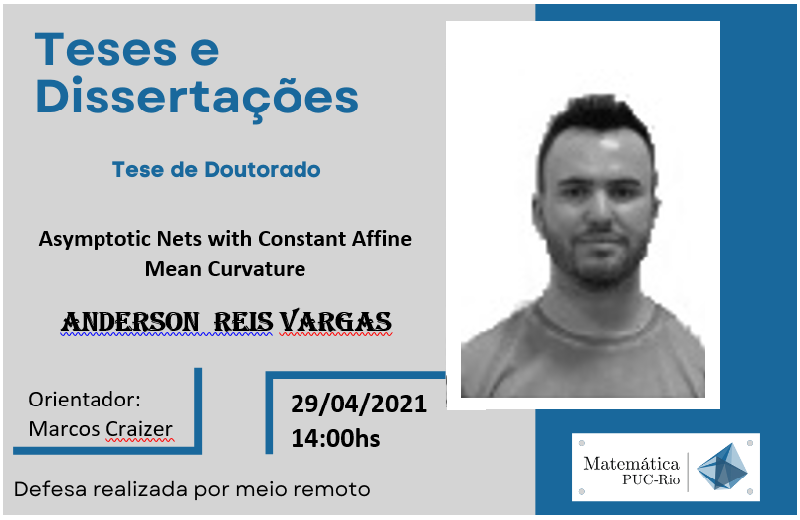Tese de Doutorado - Anderson Reis VargasAsymptotic Nets with Constant Affine Mean Curvature

Discrete Differential Geometry aims to develop a discrete theory which respects fundamental aspects of smooth theory. With this in mind, some results of smooth theory of Affine Geometry are firstly introduced since their discrete counterparts shall be treated a posteriori. The first goal of this work is to construct a discrete affine structure for nets with indefinite Blaschke metric and asymptotic parameters. For this purpose, one defines a conormal vector field , which satisfies Lelieuvre’s equations and it is associated with a real parameter ; and an affine normal vector field , which defines the cubic form of the net and makes the structure well defined. Settled the structure down, one presents the discrete affine minimal surfaces which have a beautiful geometrical characterization.
This structure also allow to study ruled surfaces with emphasis on improper affine spheres, which are proved to be equiaffinely congruent to the graph of , for some real function . Moreover, it is proposed a definition for singularities in the case of discrete improper affine spheres from the center- chord construction. Another goal here is to propose a definition for an asymptotic net with constant affine mean curvature (CAMC), in a way that encompasses discrete affine minimal surfaces and discrete affine spheres. This work is completed with the main result about a discrete version of Cayley surfaces, which are ruled improper affine spheres that can be characterized by the induced connection as: an asymptotic net with CAMC is equiaffinely congruent to a Cayley surface if and only if the cubic form C does not vanish and the affine induced connection is parallel.